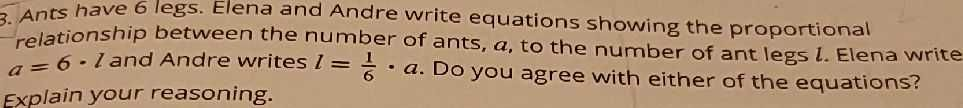### ¿Todavía tienes preguntas de matemáticas?

Pregunte a nuestros tutores expertos
Algebra
Pregunta3. Ants have $$6$$ legs. Elena and Andre write equations showing the proportional relationship between the number of ants, $$a$$ , to the number of ant legs $$l$$ . Elena write $$a = 6 \cdot l$$ and Andre writes $$l = \frac { 1 } { 6 } \cdot a$$ . Do you agree with either of the equations? Explain your reasoning.

$$l= 6a$$